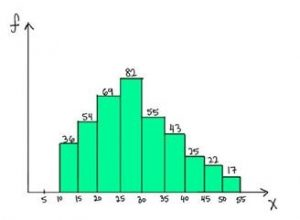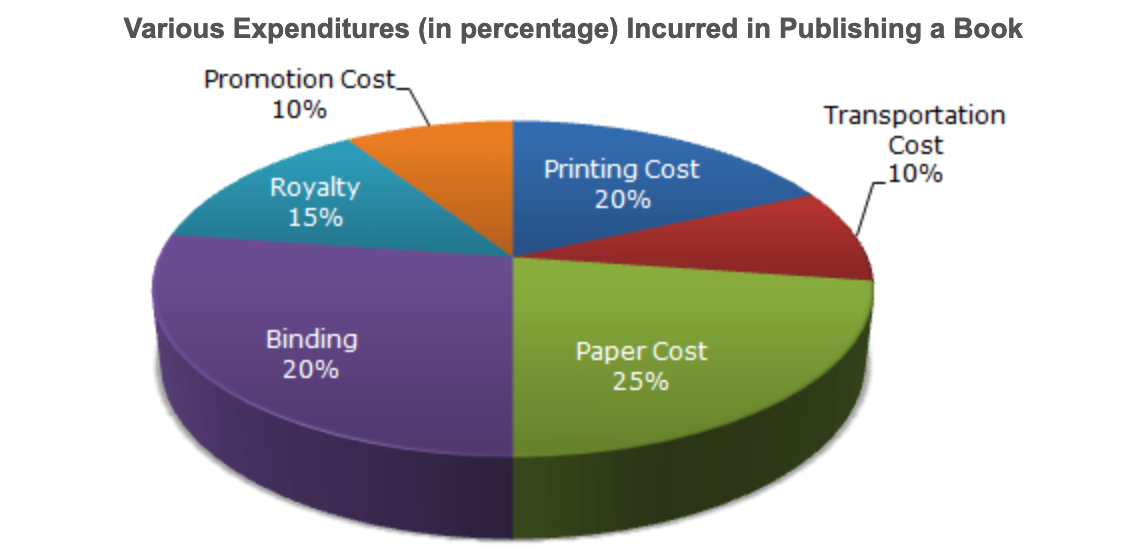Home » Business Analytics Assessment Test

The mean of 20 numbers is 18. If 3 is added to the first 10 numbers, then what is the new mean?

Out of 200 fish in an aquarium 99% are red. How many red fishes should be removed to reduce the percent of red fish to 98%?

What is the probability of getting a sum 9 from two throws of a dice?

Two cars cover the same distance at the speed of 60 and 64 kmph respectively. Find the distance traveled by them if the slower car takes 1 hour more than the faster car.

Which of the following is a possible value for the median of the below distribution?A test is administered annually. The test has a mean score of 150 and a standard deviation of 20. If Ravi’s z-score is 1.50, what was his score on the test?

The following pie-chart shows the percentage distribution of the expenditure incurred in publishing a book. Study the pie-chart and answer the questions based on it:If for a certain quantity of books, the publisher has to pay Rs. 36,000 as printing cost, then what will be the amount of royalty to be paid for these books?

Thank you for taking our survey.